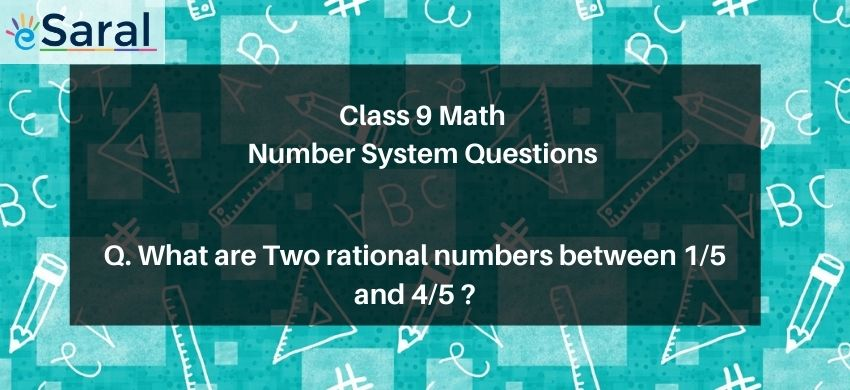Most Affordable JEE | NEET | 8,9,10 Preparation by Kota's Top IITian Doctor Faculties

# What are two rational numbers between $\frac{1}{5}$ and $\frac{4}{5}$ ?`

# Question : Two rational numbers between $\frac{1}{5}$ and $\frac{4}{5}$ are :

1. 1 and $\frac{3}{5}$
2. $\frac{2}{5}$ and $\frac{3}{5}$
3. $\frac{1}{2}$ and $\frac{2}{1}$
4. $\frac{3}{5}$ and $\frac{6}{5}$
Solution :
The correct option is 2. $\frac{2}{5}$ and $\frac{3}{5}$
Since the denominator of both rational numbers are same. So, for getting the rational numbers between the given rational numbers, we only have to consider the numerators of the rational numbers.
Two numbers between 1 & 4 are 2 and 3.
So, two rational numbers between the given rational numbers will be $\frac{2}{5}$ and $\frac{3}{5}$
So, the correct answer is option 2.